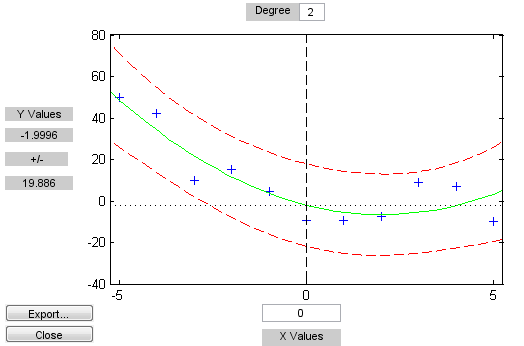polytool

Interactive polynomial fitting

Syntax

polytool(x,y)
polytool(x,y,n)
polytool(x,y,n,alpha)
polytool(x,y,n,alpha,xname,yname)
h = polytool(...)

Description

polytool(x,y) fits a line to the vectors x and y and displays an interactive plot of the result in a graphical interface. You can use the interface to explore the effects of changing the parameters of the fit and to export fit results to the workspace.

polytool(x,y,n) initially fits a polynomial of degree n. The default is 1, which produces a linear fit.

polytool(x,y,n,alpha) initially plots 100(1 - alpha)% confidence intervals on the predicted values. The default is 0.05 which results in 95% confidence intervals.

polytool(x,y,n,alpha,xname,yname) labels the x and y values on the graphical interface using xname and yname. Specify n and alpha as [] to use their default values.

h = polytool(...) outputs a vector of handles, h, to the line objects in the plot. The handles are returned in the degree: data, fit, lower bounds, upper bounds.

Examples

Interactive polynomial fitting

This example shows how to start an interactive fitting session with polytool.

rng('default') % for reproducibility
x = -5:5;
y = x.^2 - 5*x - 3 + 5*randn(size(x));

Fit a quadratic (degree-2) model with 0.90 confidence intervals.

n = 2;
alpha = 0.1;
polytool(x,y,n,alpha)